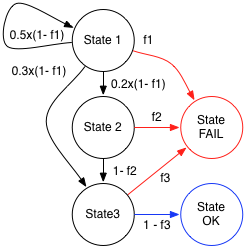Thursday, February 9, 2012

Markov-based Reliability Models. Part 2.

Let me continue with the Markov-based reliability models. This post discusses how the system-level reliability can be estimated with the help of control flow analysis. Roger C. Cheung  from the Bell Labs published one of the first articles devoted to this idea in 1980: "A USER-ORIENTED SOFTWARE RELIABILITY MODEL". In this post I would like to discuss this concept using a simple example.

Assume that we have a system of three modules as it shown in Figure A. R. Cheung has used the term "modules", however, it can be any other software executable elements like methods, functions, services, or even activities of hardware. The key point: is that we know the control flow structure.

Control flow of this example is rather simple: There is a decision block after the module 1 that defines witch of the modules will be executed next. Assume that we know the preliminary operational profile of this system: After execution of Module 1 in 5 cases of 10 the control will return to module 1, in 3 cases it will be passed to the module 3, and in 2 cases the module 2 will be executed. This information makes it possible to define the control flow of this system as a discrete time Markov chain. The state graph of this chain is shown in Figure B. Each node of this graph represents the execution of the corresponding module. This Markovian representation allows numerical computation of the probabilities of execution of each module. However, even without this computation we can see that Module 1 can be executed  several times, Module 2 will be executed only with the probability of 0.2, and Module 3 will be executed exactly one time, during the execution of the entire system.Figure B Figure C

The modules are considered to be faulty, and the faults can be activated during the execution of the modules. It is assumed that a fault activation results in the immediate failure of the system, as well as that we know the fault activation probabilities for each module: f1, f2, and f3 respectively.

The final goal is to compute the probability that the system will not fail during its execution. It is obvious that we have to consider two aspects, the probability of execution and the probability of fault activation for each module.

Cheung proposes to transform the original state graph into the one shown in the Figure C. In oder to do that, we add two additional states, "OK" and "FAIL". After that, we add an arc from the node "State 3" to the node "OK", weighted with the probability 1-f3. This arc defines successful completion of system execution. Also, we add 3 arcs from each node of the original graph to the node "FAIL". These arcs are weighted with the corresponding probabilities of fault activation, f1, f2, and f3. The weights of the rest outgoing arcs are recomputed using the original proportion (see Figure C).

This new generated graph describes utilization of the system. It starts with the execution of the module 1 and ends with successful execution of the module 3 (State OK) or with a system failure (State FAIL). The mathematical framework of the absorbing discrete time Markov chains enables the computation of the probabilities of process completion in one of these two states (see this book chapter for details). It means that the probability that the process will end in "State OK" is exactly the desired reliability of this system.

In conclusion, I want to say that this model describes the most simple way of reliability estimation with respect to the system control flow and contains strong assumptions and restrictions such as:

1) System control ﬂow must satisfy the Markov property: Future states of the process depend only upon the present state, not on the sequence of previous events.
2) Fault activations in each module are considered to be independent events.
3) A fault activation results in immediate system failure.

and so on..

Nevertheless, keep in mind that it was presented in 1980! Nowadays, analysts use this idea to create more advanced reliability models that consider e.g. continues nature of system execution, conditional probabilities of fault activation, error propagation phenomena, and many other important factors.